# Find the general solution to

(a) Find the general solution to y''-10y'+25y=0. Enter your answer as y = .... In your answer, use c1 and c2 to denote arbitrary constants and x the independent variable. Enter c1 as c1 and cas c2.

(b) Find the solution that satisfies the initial conditions y(0)=4 and y'(0)=0.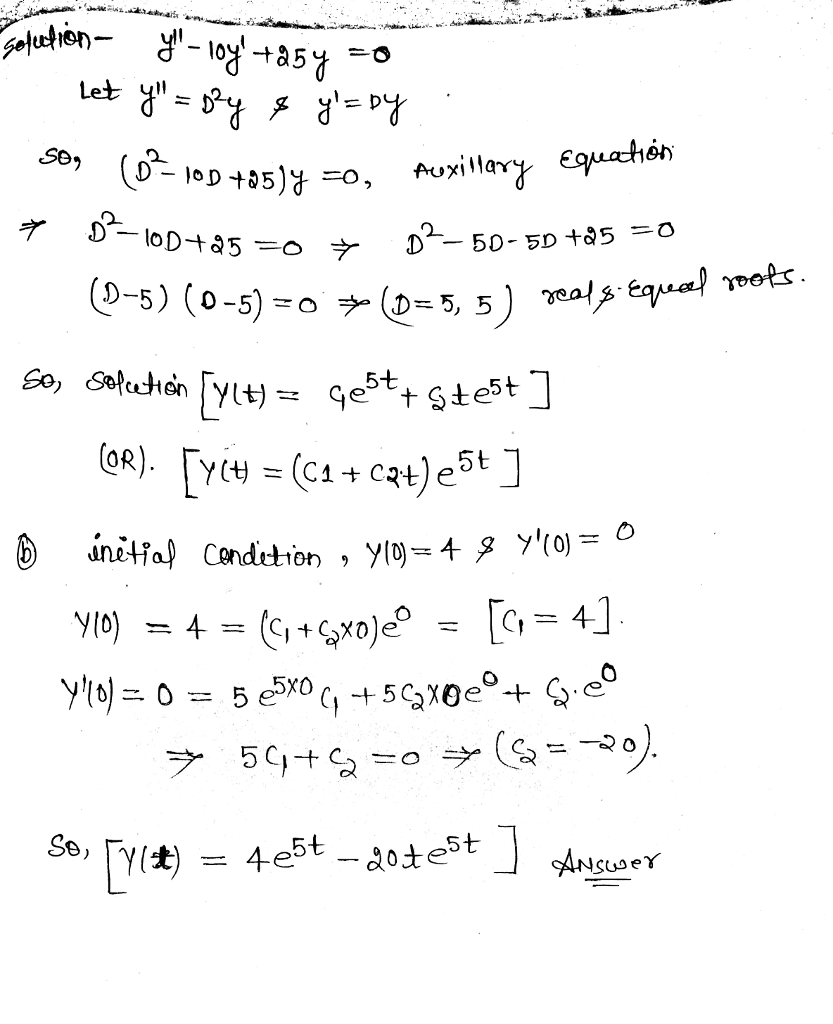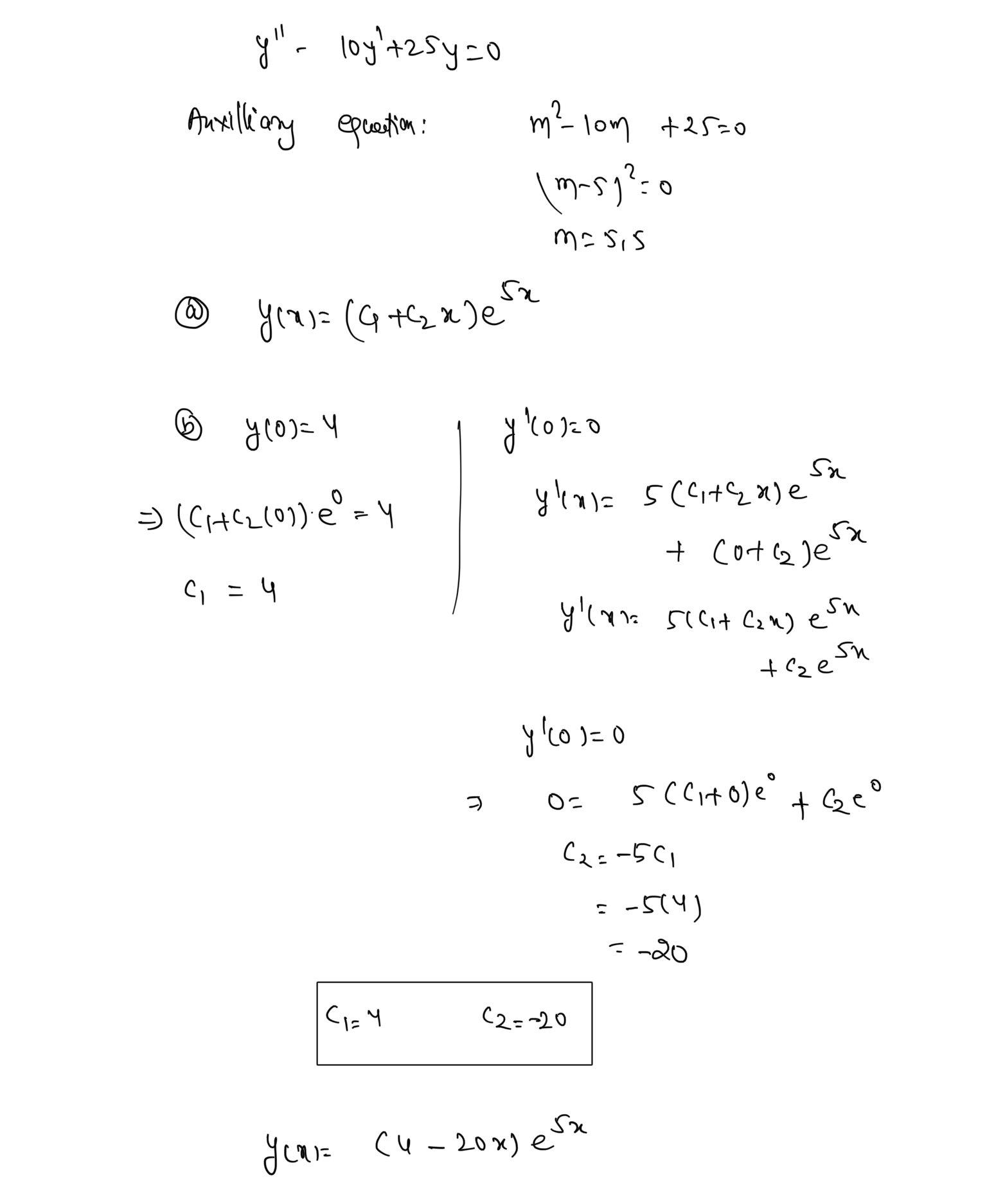#### Earn Coin

Coins can be redeemed for fabulous gifts.

Similar Homework Help Questions
• ### (3 points) (a) Find the general solution to y′′+2y′=0. Give your answer as y=... . In...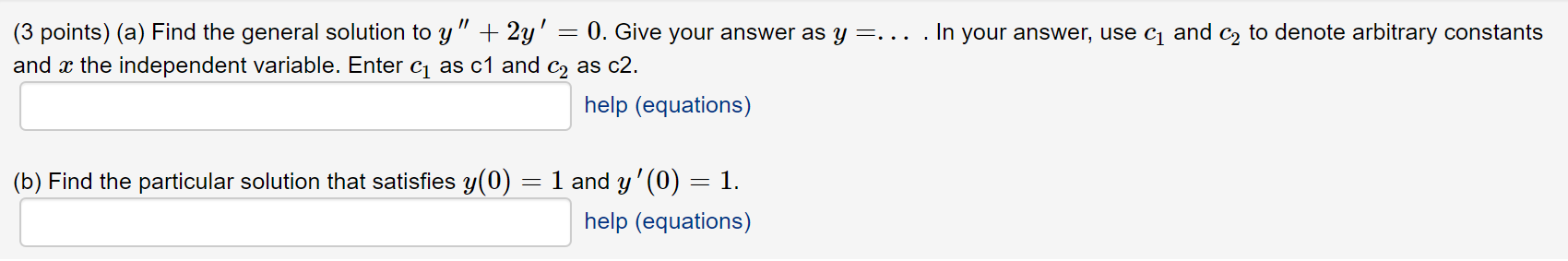(3 points) (a) Find the general solution to y′′+2y′=0. Give your answer as y=... . In your answer, use c1 and c2 to denote arbitrary constants and x the independent variable. Enter c1 as c1 and c2 as c2. (3 points) (a) Find the general solution to y" + 2y' = 0. Give your answer as y=... . In your answer, use c1 and c2 to denote arbitrary constants and x the independent variable. Enter cı as c1 and C2...

• ### (5 points) Find the general solution to the differential equation y" – 2y + 17y=0. In...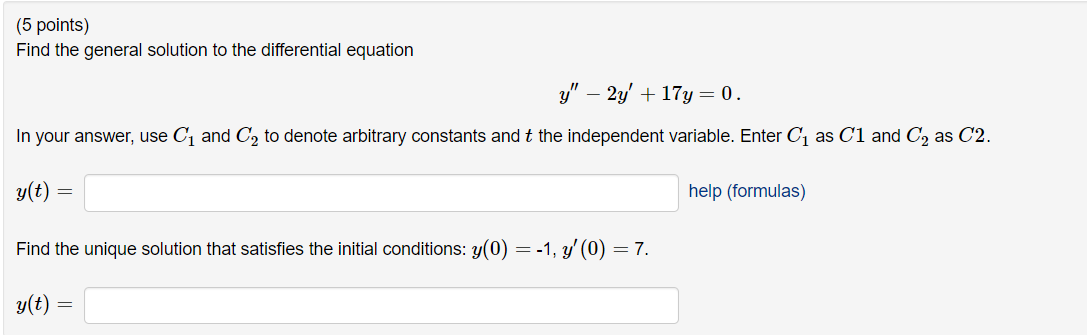(5 points) Find the general solution to the differential equation y" – 2y + 17y=0. In your answer, use Cį and C2 to denote arbitrary constants and t the independent variable. Enter Cų as C1 and C2 as С2. y(t) = help (formulas) Find the unique solution that satisfies the initial conditions: y(0) = -1, y'(0) = 7. y(t) =

• ### 1 point) (a) Find the general solution to y" +7y'-0. Give your answer as y -.....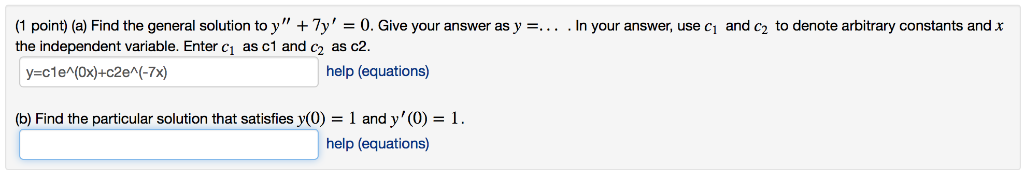1 point) (a) Find the general solution to y" +7y'-0. Give your answer as y -.. . In your answer, use ci and c2 to denote arbitrary constants and x the independent variable. Enter ci as c1 and c as c2 help (equations) (b) Find the particular solution that satisfies y(0) 1 and y'(0)1 help (equations)

• ### The answer above is NOT correct. (1 point) Find the general solution to y(4) – 8y""...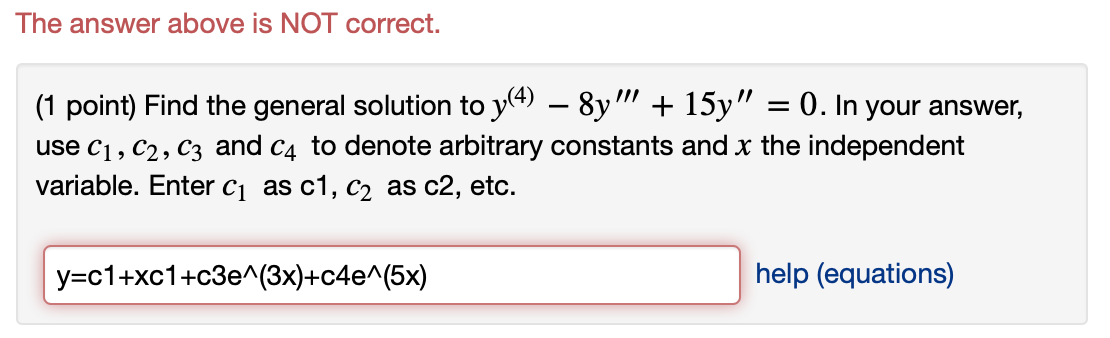The answer above is NOT correct. (1 point) Find the general solution to y(4) – 8y"" + 15y" = 0. In your answer, use C1,C2,C3 and C4 to denote arbitrary constants and x the independent variable. Enter ci as c1, c2 as c2, etc. y=c1+xc1+c3e^(3x)+c4e^(5x) help (equations)

• ### Find the general solution to y'' + 8y' + 41y = 0

Find the general solution to y'' + 8y' + 41y = 0. Give your answer as y=.... In your answer, use c1 and c2 to denote arbitrary constants and the independent variable.

• ### Assignment 9: Problem 1 Previous Problem List Next (1 point) Find the general solution to y(4)...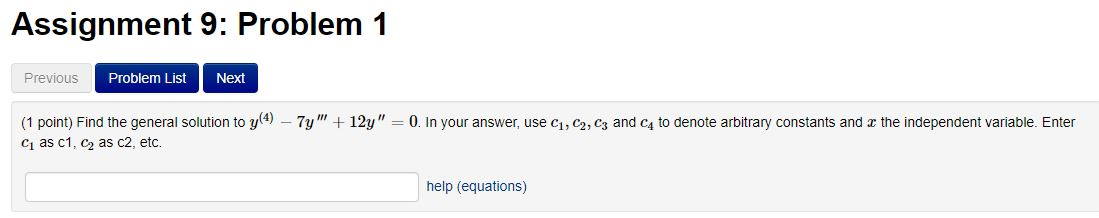Assignment 9: Problem 1 Previous Problem List Next (1 point) Find the general solution to y(4) - 7y" + 12y" = 0. In your answer, use C1, C2, C3 and C4 to denote arbitrary constants and r the independent variable. Enter C as C1, C2 as c2, etc. help (equations)

• ### (1 point) a. Find a particular solution to the nonhomogeneous differential equation y" + 3y -...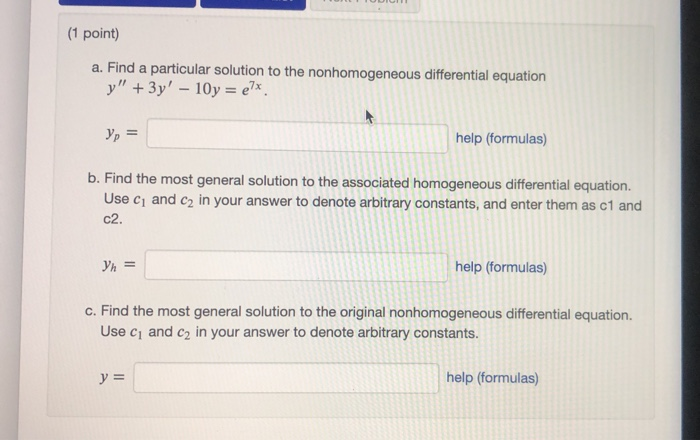(1 point) a. Find a particular solution to the nonhomogeneous differential equation y" + 3y - 10y = ex. yp = help (formulas) b. Find the most general solution to the associated homogeneous differential equation. Use cy and c2 in your answer to denote arbitrary constants, and enter them as c1 and c2. Yh = help (formulas) c. Find the most general solution to the original nonhomogeneous differential equation. Use cy and C2 in your answer to denote arbitrary constants....

• ### Consider the differential equation: y' - 5y = -2x – 4. a. Find the general solution...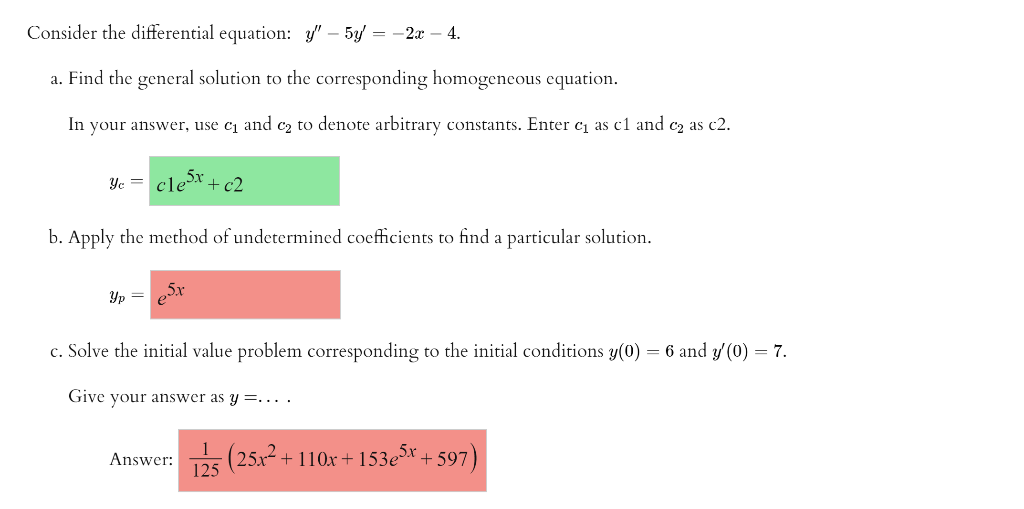Consider the differential equation: y' - 5y = -2x – 4. a. Find the general solution to the corresponding homogeneous equation. In your answer, use cı and ca to denote arbitrary constants. Enter ci as c1 and ca as c2. Yc = cle cle5x - + c2 b. Apply the method of undetermined coefficients to find a particular solution. yp er c. Solve the initial value problem corresponding to the initial conditions y(0) = 6 and y(0) = 7. Give...

• ### (1 point) Find the general solution to y + 8y" + 25y' = 0. In your...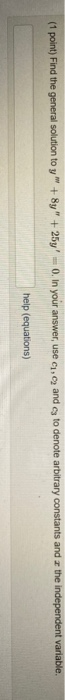(1 point) Find the general solution to y + 8y" + 25y' = 0. In your answer, use c. and.cy to denote arbitrary constants and the independent variable. help (equations)

• ### (5 points) a. Find a particular solution to the nonhomogeneous differential equation y" + 4y =...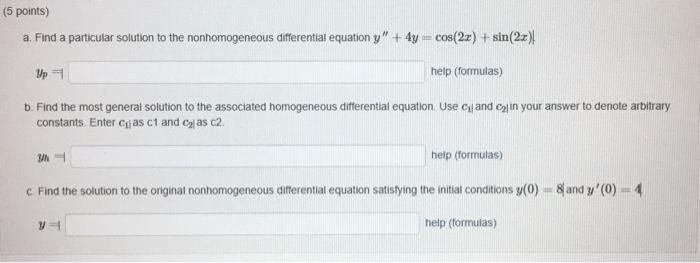(5 points) a. Find a particular solution to the nonhomogeneous differential equation y" + 4y = cos(2x) + sin(2x) Up help (formulas) b. Find the most general solution to the associated homogeneous differential equation. Use cand in your answer to denote arbitrary constants. Enter Cas c1 and ca as c2 Wh help (formulas) c. Find the solution to the original nonhomogeneous differential equation satisfying the initial conditions y(0) = 8 and y'(0) = 4 y help (formulas)